# APPROX-MIC Implementation Details¶

The core implementation of libmine is built from scratch in ANSI C starting from the pseudocode provided in DOI: 10.1126/science.1205438, Supplementary On-line Material (SOM), as no original Java source code is available. The level of detail of the pseudocode leaves a few ambiguities and in this section we list and comment the most crucial choices we adopted for the algorithm steps whenever no explicit description was provided. Obviously, our choices are not necessarily the same as in the original Java version (MINE.jar, http://www.exploredata.net/). The occurring differences can be ground for small numerical discrepancies as well as for difference in performance (DOI: 10.1093/bioinformatics/bts707).

1. In SOM, Algorithm 5, the characteristic matrix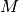is computed in the loop starting at line 7 for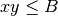. This is in contrast with the definition of the MINE measures (see SOM, Sec. 2) where the corresponding bound is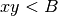for all the four statistics. We adopted the same bound as in the pseudocode, i.e..

2. The MINE statistic MCN is defined as follows in SOM, Sec. 2: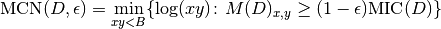As for MINE.jar (inferred from Table S1), we set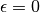and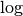to be in base 2. Finally, as specified in Point 1 above, we use the boundas in the SOM pseudocode rather than theas in the definition. This led to implement the formula: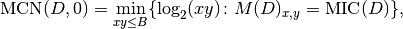being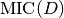the maximum value of the matrix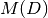.

3. In EquipartitionYAxis() (SOM, Algorithm 3, lines 4 and 10), two ratios are assigned to the variable desiredRowSize, namely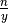and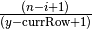. We choose to consider the ratios as real numbers; a possible alternative is to cast desiredRowSize to an integer. The two alternatives can give rise to different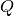maps, and thus to slightly different numerical values of the MINE statistics.

4. In some cases, the function EquipartitionYAxis() can return a mapwhose number of clumps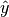is smaller than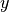, e.g. when in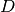there are enough points whose second coordinates coincide. This can lead to underestimate the normalized mutual information matrix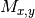(SOM, Algorithm 5, line 9), whereis obtained by dividing the mutual information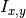for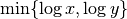. To prevent this issue, we normalize instead by the factor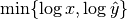.

5. The function GetClumpsPartition(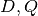) is discussed (SOM page 12), but its pseudocode is not explicitely available. Our implementation is defined here in GetClumpsPartition() algorithm. The function returns the map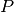defining the clumps for the set, with the constraint of keeping in the same clump points with the same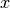-value.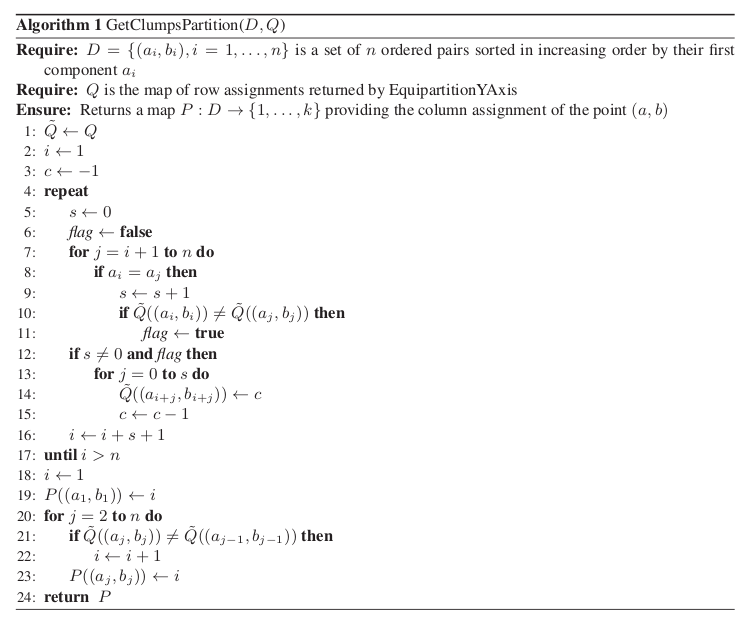GetClumpsPartition() algorithm

6. We also explicitly provide the pseudocode for the GetSuperclumpsPartition() function (SOM page 13) in GetSuperclumpsPartition() algorithm. This function limits the number of clumps when their number k is larger than a given bound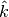. The function calls the GetClumpsPartition() and, for math:k>hat{k} it builds an auxiliary set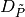as an input for the EquipartitionYAxis function discussed above (Points 3-4).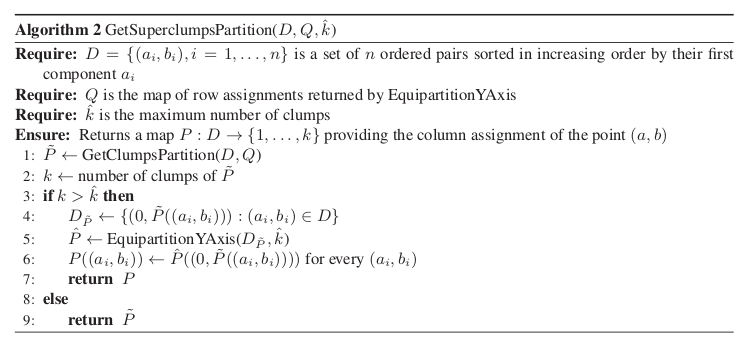GetSuperclumpsPartition() algorithm

7. We observed that the GetSuperclumpsPartition() implemented in MINE.jar may fail to respect theconstraints on the maximum number of clumps and a mapwith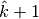superclumps is actually returned. As an example, the MINE.jar applied in debug mode (d=4 option) with the same parameters (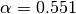,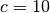) used in the original work to the pair of variables (OTU4435,OTU4496) of the Microbioma dataset, returns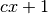clumps, instead of stopping at the bound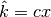for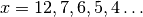.

8. The possibly different implementations of the GetSuperclumpsPartition() function described in Points 6-7 can lead to minor numerical differences in the MIC statistics. To confirm this effect, we verified that by reducing the number of calls to the GetSuperclumpsPartition() algorithm, we can also decrease the difference between MIC computed by minepy and by MINE.jar, and they asymptotically converge to the same value.

9. In our implementation, we use double-precision floating-point numbers (double in C) in the computation of entropy and mutual information values. The internal implementation of the same quantities in MINE.jar is unknown.

10. In order to speed up the computation of the MINE statistics, we introduced two improvements (with respect to the pseudo-code), in OptimizeXAxis(), defined in Algorithm 2 in SOM):

• Given a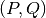grid, we precalculate the matrix of number of samples in each cell of the grid, to speed up the computation of entropy values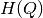,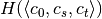,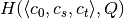and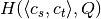.
• We precalculate the entropy matrix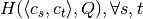to speed up the computation of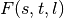(see Algorithm 2, lines 10–17 in SOM).

These improvements do not affect the final results of mutual information matrix and of MINE statistics.